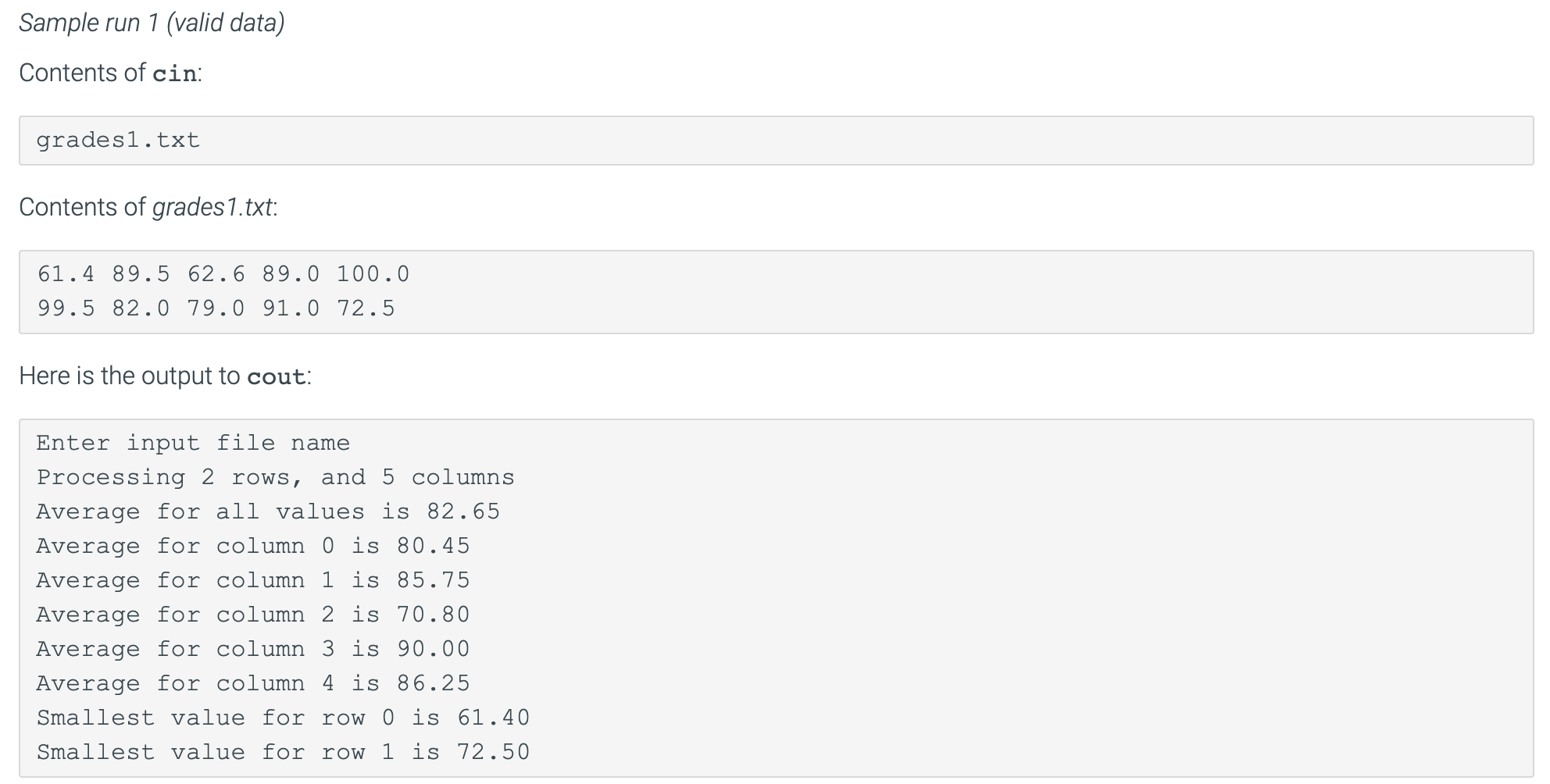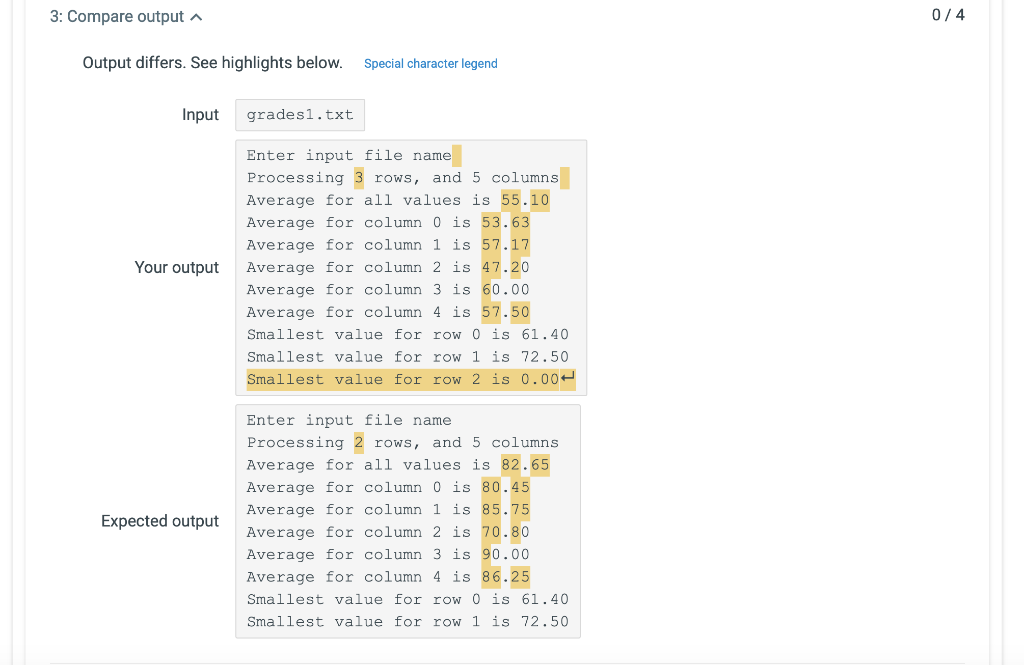# (Solved) : Sure Modify Code Determine 2 Rows Instead 3 Without Affecting Outputs Posted Sample Output Q42768454 . . .

I am not sure how to modify my code for it to determine 2 rowsinstead of 3 without not affecting my other outputs. I have postedthe sample output , my code and the output I am receiving.

CODE:

#include <iostream>

#include <iomanip>

#include <string>

#include <fstream>

using namespace std;

const int MAX_COLUMNS = 5;

{

//variable declaration

int i, j;

string line;

//Openin the file

ifstream fin;

fin.open(inputFileName.c_str(),ios::in);

//Check file exists or not

if (fin.fail())

{

//Failed to open file

return -1;

}

else

{

for (i = 0; i<maxRows && fin.good();i++)

{

for (j = 0; j<MAX_COLUMNS; j++)

{

//Read the value from each row

fin >> values[i][j];

//Return 0 if the file contains no data

if (fin.fail() && i == 0)

{

return 0;

}

}

}

}

//Close the file

fin.close();

//Return the number of rows read

return i;

}

//Avearge function calcuates the average of the array

double average(doublevalues[][MAX_COLUMNS], int numberRows)

{

double sum = 0;

int i, j;

//Iterate over rows

for (i = 0; i<numberRows; i++)

{

//Iterate over columns

for (j = 0; j<MAX_COLUMNS; j++)

{

//Calculate the sum

sum = sum + values[i][j];

}

}

//Find the avearge and return it

return (sum /(double)(numberRows*MAX_COLUMNS));

}

//columnAverage function calculates the average of eachcolumn

double columnAverage(doublevalues[][MAX_COLUMNS], int column,int numberRows)

{

double sum = 0;

int i;

//Iterate over rows

for (i = 0; i<numberRows; i++)

{

//Calculate the sum

sum += values[i][column];

}

//Find the avearge and return it

return (sum /(double)(numberRows));

}

//smallestValue function returns the smallest value in eachrow

double smallestValue(doublevalues[][MAX_COLUMNS], int rowNumber)

{

double minVal = values[rowNumber];

int i;

//Iterate over columns

for (i = 0; i<MAX_COLUMNS; i++)

{

//Calculate the sum

if (values[rowNumber][i] < minVal)

{

//Update the minimum value

minVal = values[rowNumber][i];

}

}

//Return min value

return minVal;

}

//Main function

int main()

{

int rows, cols;

const int MAX_ROWS = 30;

string inputFileName;

int actualRows;

//Set to two decimal places

cout << fixed << setprecision(2);

cout << “Enter input file name “;

cin >> inputFileName;

//Check number of rows

if (actualRows == -1)

{

//Print error message

cout << endl << “File “” << inputFileName<< “” could not be opened ” << endl;

}

else if (actualRows == 0)

{

//Print error message

cout << endl << “The input file “” <<inputFileName << “” did not contain any data ” <<endl;

}

else

{

cout << “nProcessing ” << actualRows << “rows, and ” << MAX_COLUMNS << ” columns ” <<endl;

//Print average value

cout << “Average for all values is ” <<average(grades, actualRows) << endl;

//Print column wise average

for (cols = 0; cols < MAX_COLUMNS;cols++)

{

//Print column wise average

cout << “Average for column ” << cols << ” is” << columnAverage(grades, cols, actualRows) <<endl;

}

//Print row wise smallest value

for (rows = 0; rows < actualRows;rows++)

{

//Print row wise smallest value

cout << “Smallest value for row ” << rows << “is ” << smallestValue(grades, rows) << endl;

}

}

return 0;

}Sample run 1 (valid data) Contents of cin grades1.txt Contents of grades1.txt: 61.4 89.5 62.6 89.0 100.0 99.5 82.0 79.0 91.0 72.5 Here is the output to cout: Enter input file name Processing 2 rows, and 5 columns Average for all values is 82.65 Average for column 0 is 80.45 Average for column 1 is 85.75 Average for column 2 is 70.80 Average for column 3 is 90.00 Average for column 4 is 86.25 Smallest value for row 0 is 61.40 Smallest value for row 1 is 72.50 3: Compare output 0/4 Output differs. See highlights below. Special character legend Input grades1.txt Enter input file name Processing 3 rows, and 5 columns Average for all values is 55.10 Average for column 0 is 53.63 Average for column 1 is 57.17 Your output Average for column 2 is 47.20 60.00 Average for column 3 Average for column 4 is 57.50 Smallest value for row 0 is 61.40 Smallest value for row 1 is 72.50 Smallest value for row 2 is 0.00 Enter input file name Processing 2 rows, and 5 columns Average for all values is 82.65 Average for column 0 is 80.45 85.75 Average for column 1 Expected output Average for column 2 is 70.80 90.00 Average for column 3 Average for column 4 is 86.25 Smallest value for row 0 is 61.40 Smallest value for row 1 is 72.50 Show transcribed image text Sample run 1 (valid data) Contents of cin grades1.txt Contents of grades1.txt: 61.4 89.5 62.6 89.0 100.0 99.5 82.0 79.0 91.0 72.5 Here is the output to cout: Enter input file name Processing 2 rows, and 5 columns Average for all values is 82.65 Average for column 0 is 80.45 Average for column 1 is 85.75 Average for column 2 is 70.80 Average for column 3 is 90.00 Average for column 4 is 86.25 Smallest value for row 0 is 61.40 Smallest value for row 1 is 72.50
3: Compare output 0/4 Output differs. See highlights below. Special character legend Input grades1.txt Enter input file name Processing 3 rows, and 5 columns Average for all values is 55.10 Average for column 0 is 53.63 Average for column 1 is 57.17 Your output Average for column 2 is 47.20 60.00 Average for column 3 Average for column 4 is 57.50 Smallest value for row 0 is 61.40 Smallest value for row 1 is 72.50 Smallest value for row 2 is 0.00 Enter input file name Processing 2 rows, and 5 columns Average for all values is 82.65 Average for column 0 is 80.45 85.75 Average for column 1 Expected output Average for column 2 is 70.80 90.00 Average for column 3 Average for column 4 is 86.25 Smallest value for row 0 is 61.40 Smallest value for row 1 is 72.50

Answer to I am not sure how to modify my code for it to determine 2 rows instead of 3 without not affecting my other outputs. I ha…

We are the best freelance writing portal. Looking for online writing, editing or proofreading jobs? We have plenty of writing assignments to handle.